Question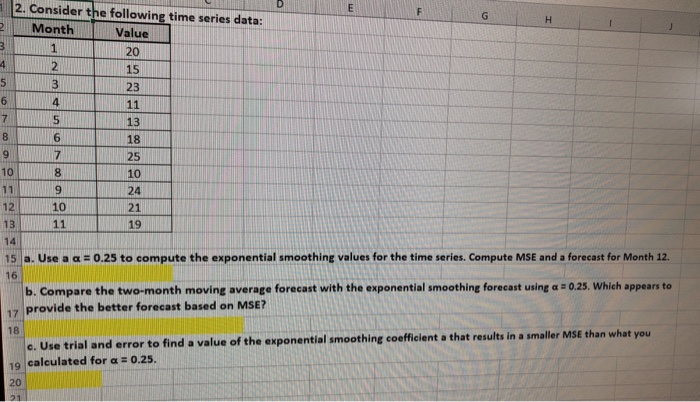2. Consider the following time series data: 2Month Value 20 15 23 6 4 13 6 18 25 10 8 10 9 24 12 10 21 13 19 14 15 la. Use a α # 0.25 to compute the exponential smoothing values for the time series. Compute MSE and a forecast for Month 12. b. Compare the two-month moving average forecast with the exponential smoothing forecast using a 0.25. Which appears to 17 provide the better forecast based on MSE? rial and error to find a value of the exponential smoothing coofficilent a that results in a smaller MSE than what you 19. calculated for α :0.25
Can you piease Show how got the answer neps me Undlerstand H better hank you So much

a. If Y(t) is the value for month t, the forecast for month t+1 using exponential smoothing model isWe will set the first month forecast as the the actual value, that is F(1) = 20. The forecast for month 2 isWe compute the 2 month moving average forecast usingFor example the forecast for month 3 is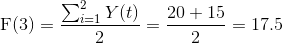Since we want to compare a 2 month moving average with exponential forecast, we will calculate the MSE (Mean Square error) using the data from 3rd month to month 11Prepare the following sheet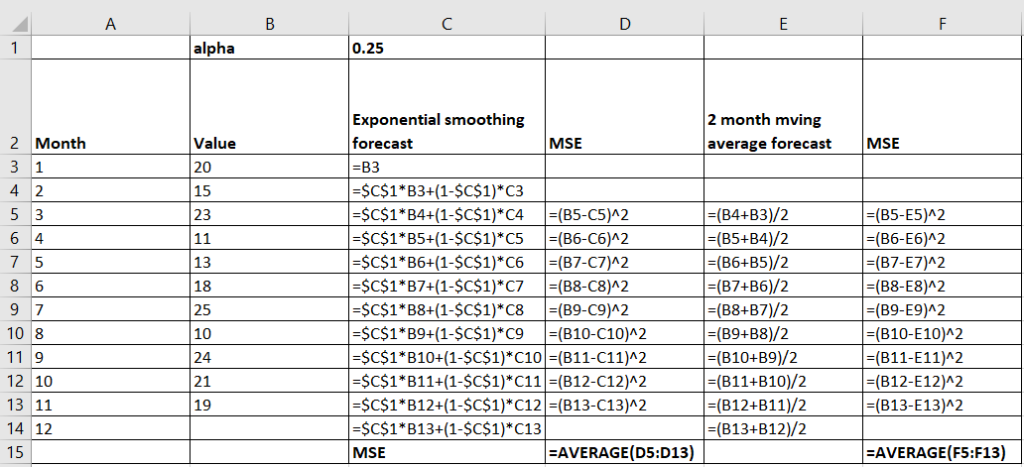We have kept the value of alpha in a separate cell so that we can do the trial and error required in part c)

get this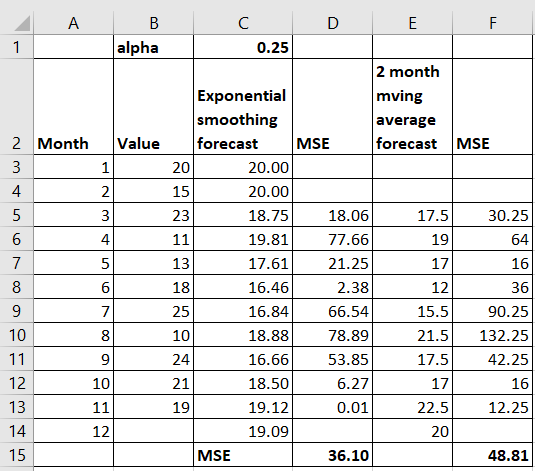a) MSE for exponential forecast with alpha=0.025 is 34.99, Forecast for the month of 12 is 19.09

b) The MSE for 2 month moving average forecast is 48.81 and it is larger than the MSE for exponential smoothing forecast. Hence we can say that exponential smoothing method provides a better forecast based on MSE

c. Now we can use trial and error by changing the value of alpha in cell C1. Try values between 0 and 1 starting from 0.01 to 1.

Or we can use the solver in excel to find the value of alpha which will give the lowest MSE.

Set up the solver using data---> solver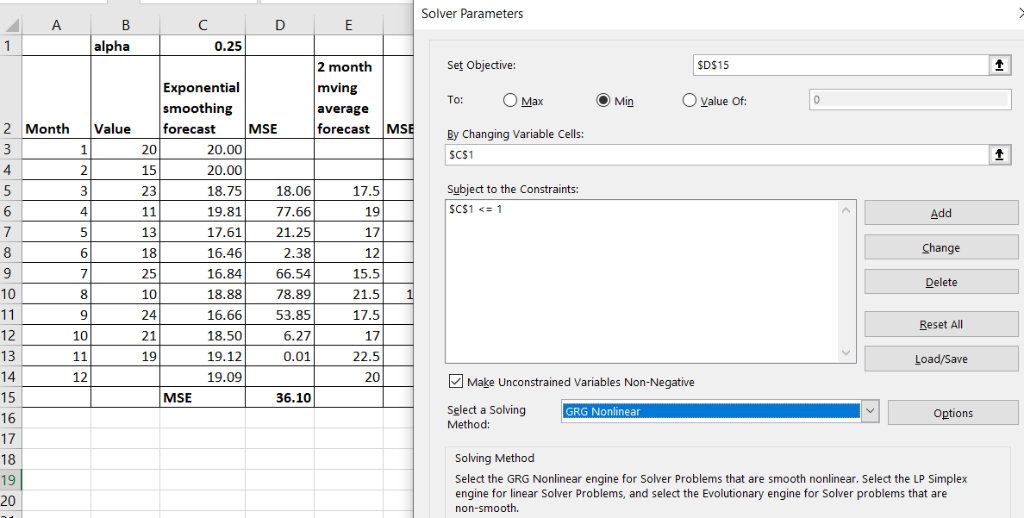get this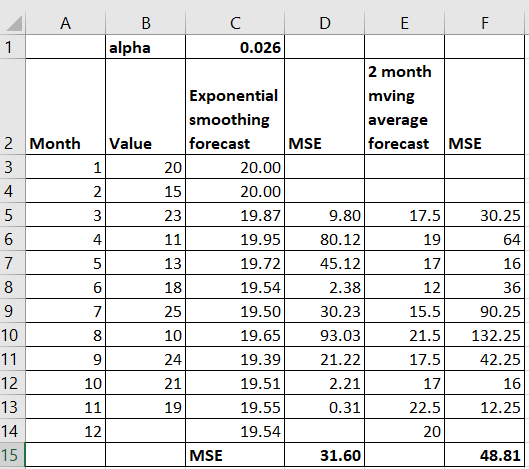We can see that for alpha=0.03, results in a smaller MSE than we calculated for alpha=0.25

#### Earn Coins

Coins can be redeemed for fabulous gifts.

Similar Homework Help Questions
• ### Omework Consider the following time series data Month 1 2 3 4 5 6 7 Value 21 14 18 13 18 21 14 a....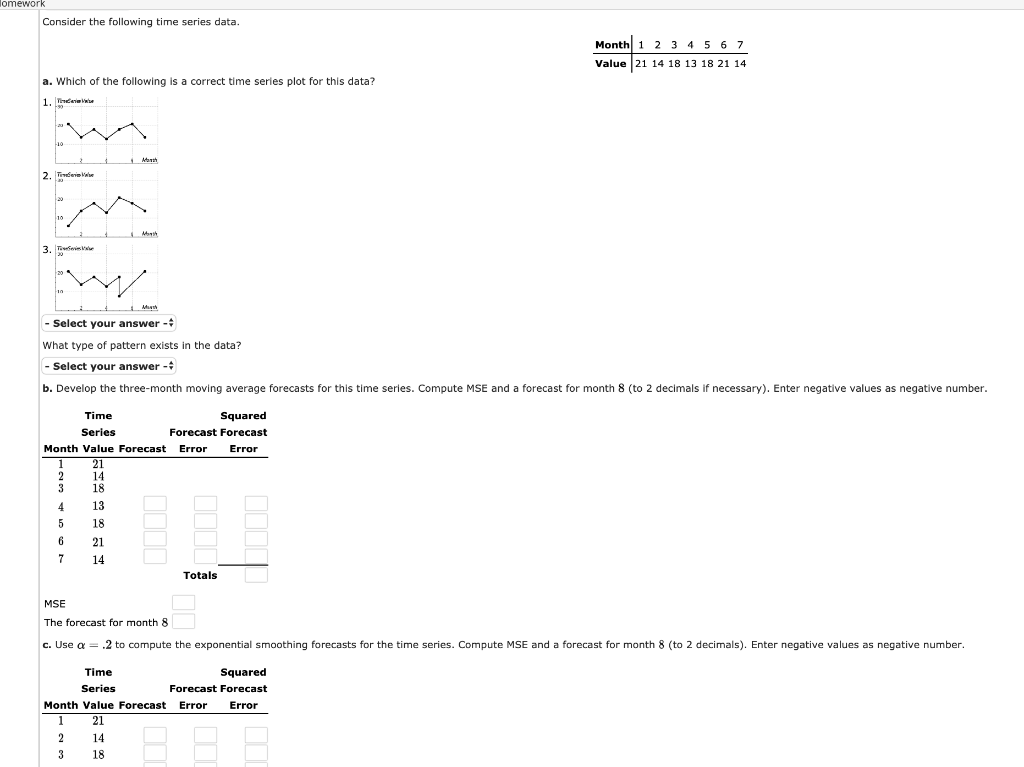omework Consider the following time series data Month 1 2 3 4 5 6 7 Value 21 14 18 13 18 21 14 a. Which of the following is a correct time series plot for this data? や" -Select your answer- What type of pattern exists in the data? -select your answer- b. Develop the three-month moving average forecasts for this time series. Compute MSE and a forecast for month 8 (to 2 decimals if necessary). Enter negative values as...

• ### Consider the following time series data. Week 1 2 3 4 5 6 Value 19 11 16 1017 15 (a) Construct a ...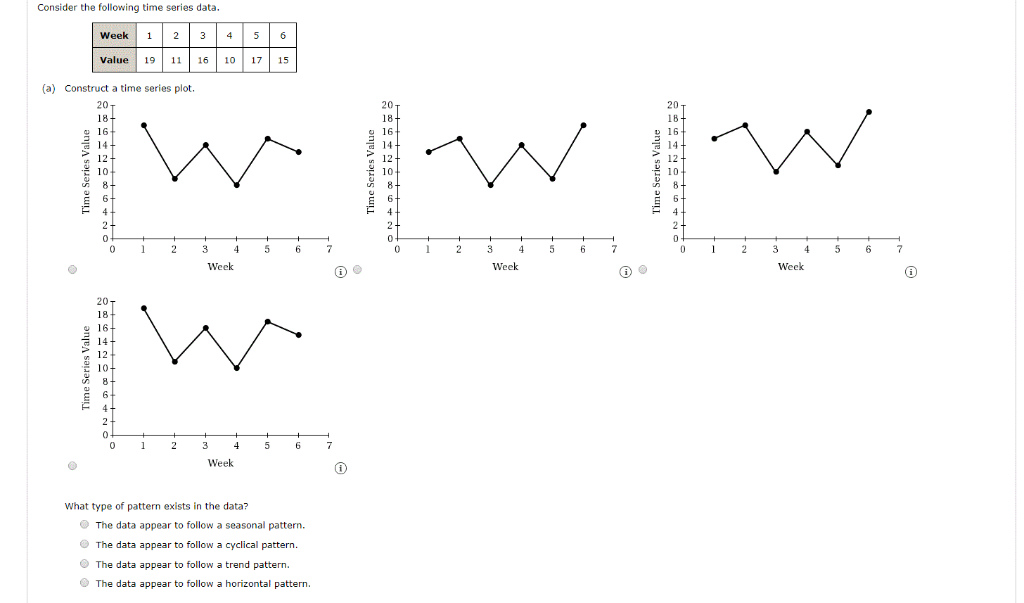Consider the following time series data. Week 1 2 3 4 5 6 Value 19 11 16 1017 15 (a) Construct a time series plot. 20 20 20 18 16 14 12 10 c 14 12 12 0 23 4 5 67 0 23 4 5 67 Week Weck Week 20 18 0 1 2345 6 7 Week What type of pattern exists in the data? The data appear to follow a seasonal pattern. The data appear to follow a...

• ### Consider the following time series data. Week 1 2 3 4 5 6 Value 19 13 16 12 18 14 a. Whic...

Consider the following time series data. Week 1 2 3 4 5 6 Value 19 13 16 12 18 14 a. Which of the following is a correct time series plot for this data? - Select your answer -plot #1plot #2plot #3Item 1 What type of pattern exists in the data? - Select your answer -VerticalHorizontalScatterItem 2 b. Develop the three-week moving average forecasts for this time series. Compute MSE and a forecast for week  (to 2 decimals if necessary)....

• ### Consider the following time series data. Week 1 2 3 4 5 6 Value 19 14...

Consider the following time series data. Week 1 2 3 4 5 6 Value 19 14 17 12 17 14 Develop a three-week moving average for this time series. Compute MSE and a forecast for week 7. Round your answers to two decimal places. Week Time Series Value Forecast 1 19 2 14 3 17 4 12 5 17 6 14 MSE: The forecast for week 7: Use = 0.2 to compute the exponential smoothing values for the time series....

• ### Please help Consider the following time series data. Week 1 N 3 4 5 6 Value...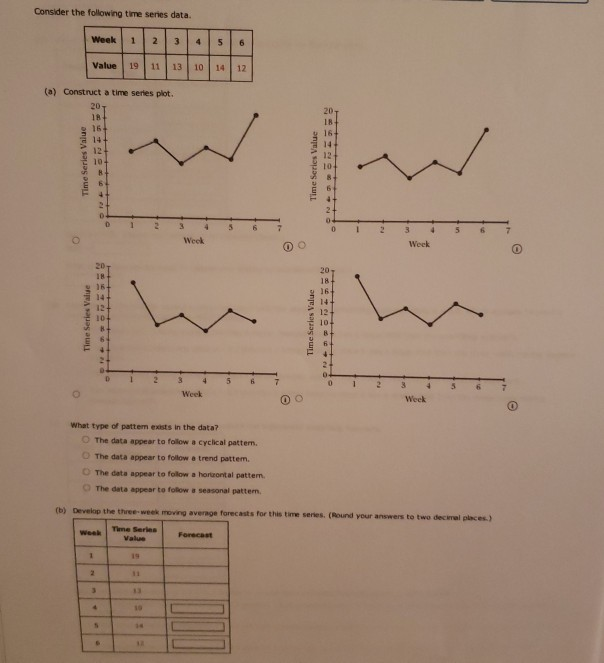Please help Consider the following time series data. Week 1 N 3 4 5 6 Value 19 11 13 10 14 12 (a) Construct a time series plot. 20 18 20 18 14 12 10 Week 3 4 Week D 20 18+ 16 Time Series Value Time Series Value 5 Week 0 Wook What type of pattem exists in the data? The data appear to follow a cyclical pattern. The data appear to follow a trend pattem. The data appear...

• ### Consider the following time series data. Week 1 2 3 4 5 6 Value 17 13...Consider the following time series data. Week 1 2 3 4 5 6 Value 17 13 15 11 15 13 (a) Choose the correct time series plot. (i) (ii) (iii) (iv) - Select your answer -Graph (i)Graph (ii)Graph (iii)Graph (iv)Item 1 What type of pattern exists in the data? - Select your answer -Horizontal PatternTrend PatternItem 2 (b) Develop a three-week moving average for this time series. Compute MSE and a forecast for week 7. If required, round your answers...

• ### Consider the following time series data Week 1 2 3 4 5 6 Value 19 14...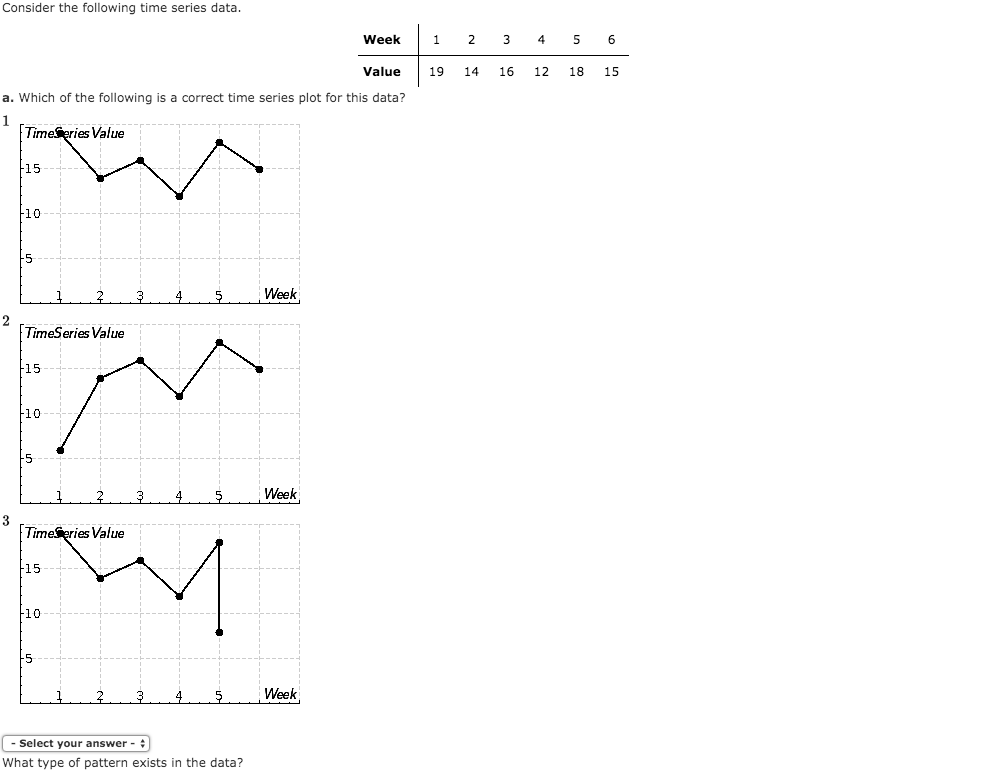Consider the following time series data Week 1 2 3 4 5 6 Value 19 14 16 12 18 15 a. Which of the following is a correct time series plot for this data? 1 Time eries Value 15 10 Week 2 TimeSeries Value 15 10 5 Week 4 3 TimeSeries Value 15 10 -5 Week 4 5 Select your answer What type of pattern exists in the data? What type of pattern exists in the data? Horizontal b. Develop...

• ### Consider the following time series data.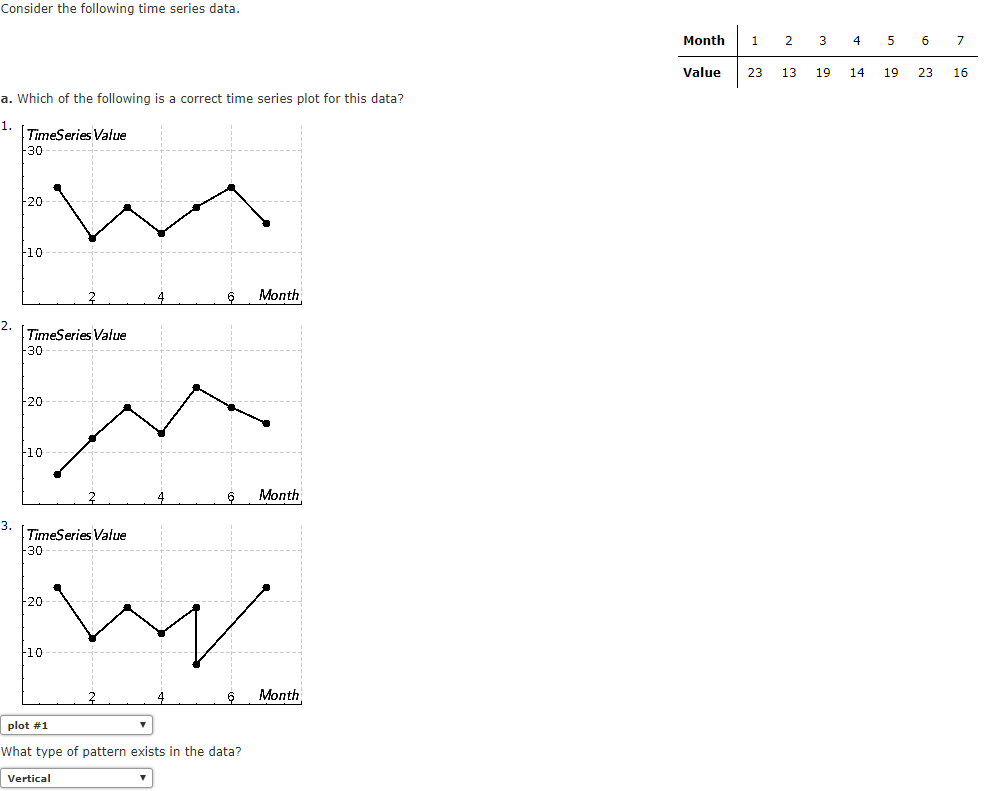Please help :) a. Which of the following is a correct time series plot for this data? b. Develop the three-month moving average forecasts for this time series. Compute MSE and a forecast for month 8 (to 2 decimals if necessary).  c. Use α-.2 to compute the exponential smoothing forecasts for the time series. Compute MSE and a forecast for month 8 (to 2 decimals). Enter negative values as negative number.  d. Compare the three-month moving average approach with the exponential smoothing approach using...

• ### Consider the following time series data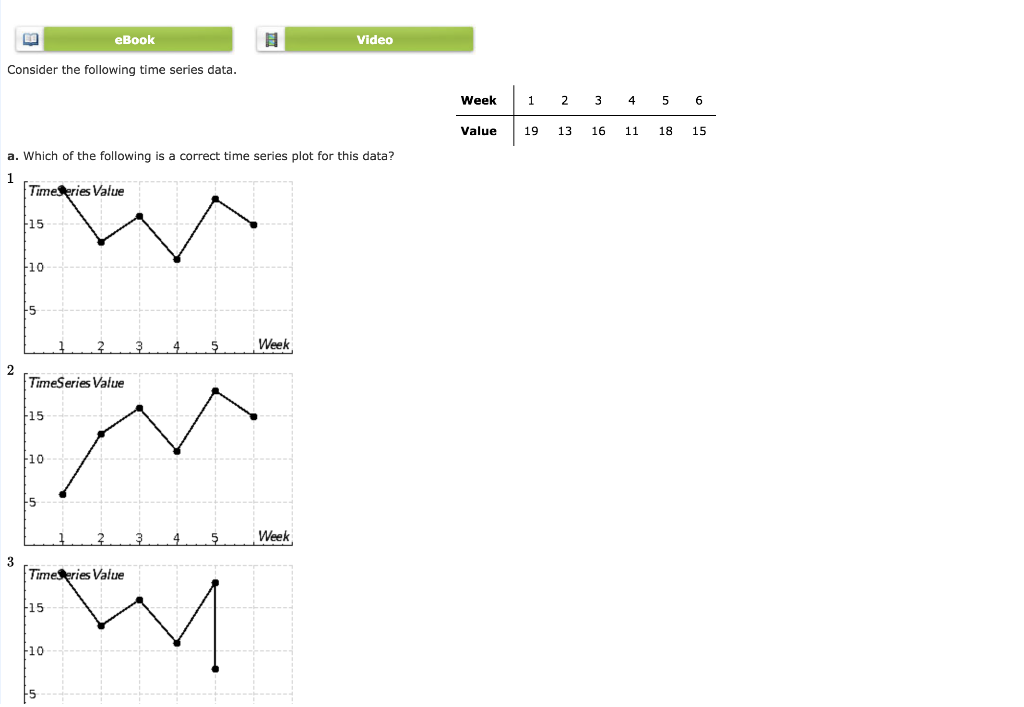Consider the following time series dataa. Which of the following is a correct time series plot for this data?b. Develop the three-week moving average forecasts for this time series. Compute MSE and a forecast for week 7 (to 2 decimals if necessary) c. Use α = .2 to compute the exponential smoothing forecasts for the time series. Compute MSE and a forecast for week 7 ( 2 decimals). d. Compare the three-week moving average approach with the exponential smoothing approach using α-.2,...